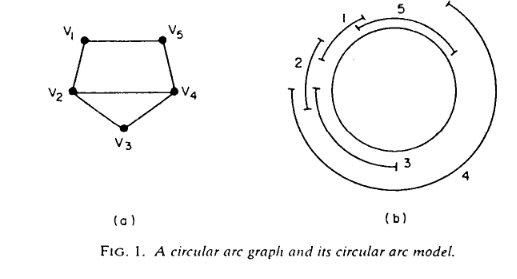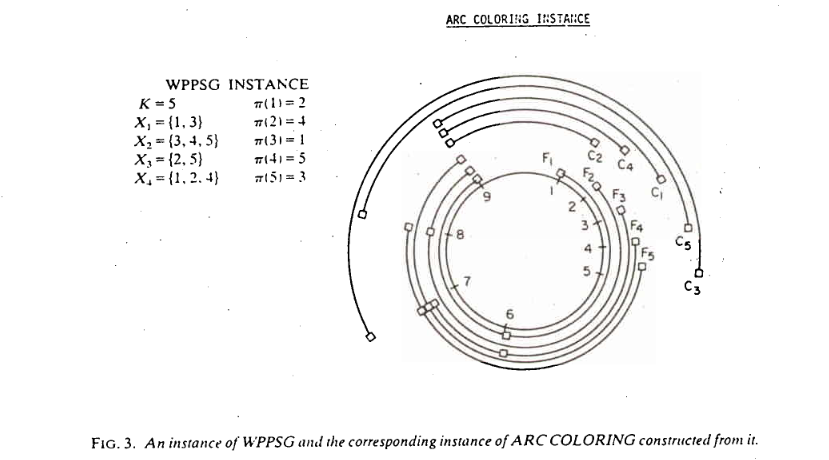# Arc Coloring

Last week we did a subproblem along the way to the next problem in the appendix.  This week we are making a second stop.

The problem: Arc Coloring.  This problem is not in the appendix.

The description: Given a circle divided into m equally spaced points, and a set {A1..An} of “arcs”.  Each arc Ai is represented by a pair (ai, bi) signifying an arc that starts at point ai and continues clockwise to point bi

A circular arc graph is a graph built from this set of arcs, with one vertex for each arc, and an edge between two arcs if they intersect at one or more points (the starting point of an interval ai does not count for overlap purposes)

The arc coloring question asks: Given an integer K, and a circular arc graph G, can we color G in K or fewer colors?  Alternately, we are asking whether we can partition the given set of arcs into K or fewer groups with no intersections within any group.

Example: The paper by Garey, Johnson, Miller, and Papadimitriou gives this example of a circular arc graph:The right side is the circle and the arcs, the left side is the graph.  So we can see there are edges connecting v1 to v2 and v5 because arc 1 overlaps with both arcs 2 and 5.

This graph can be colored with 3 colors (v2, v3, and v4 form a clique, so at least 3 colors will be needed, and coloring v1 the same color as v4 and v5 the same color of v1 will color the graph in just 3 colors).

Note that we already know that the general graph coloring problem is NP-Complete.  We are asking here whether this restricted kind of graph can be colored in polynomial time.  It will turn out that coloring this graph will be NP-Complete as well.

Reduction: The reason we talked about the Word Problem for Products of Symmetric Groups last time was to reduce from it here.

So we start with an instance of WPPSG- a collection of sets X1 through Xm, an integer K, and a goal permutation π.  We’ll assume that each number from 1 to K occurs in at least one X set (if not, if π moves that number we have an automatic “no” instance, and if it doesn’t, we can just remove the number from consideration (and shift all larger numbers down 1)).  We want to build a graph that is colorable in K colors or less if and only if our WPPSG instance is solvable.

Our circle will have K+m points on it.  Each set Xi will generate a set of arcs.  Let li be the index of the first X set that contains i, let li be the index of the second such set, and so on.  So using our example from last time, we’d say that l5 is 1 (because X1 is the first set to have a 5 in it), and l5 is 3 (because X3 is the second set to have a 5 in it).  We’ll denote the last such label as li[k(i)]

So, for each Xi we build a set of arcs:

• Ai1 = (i, K+li)
• Ai2 = (K+li, K+li)
• Ai,k(i) = (K+li[k(i)-1], K+li[k(i)])

This creates a set of arcs that are disjoint, and who cover the points from i to K+li[k(i)]

Each set Xi also creates an arc Ci = (K+li[k(i)], π-1(i)). The “π-1(i)” is just the inverse of the π permutation we started with, and tells us for each number from 1..K what position that number should end up in.  This Ci arc continues the Ai arcs from where they “end” and go around to π-1(i).

Here is an example of how this gets built from the paper:The actual proof that this conversion works is hard and complicated.  The general idea is that for each point in the circle, we need a different color for each arc that crosses the point.  The different colors can map to the permutations of the numbers from 1-K.  They show that you can build a permutation of colors that works based on the permutations you can do on the X sets, and so you can get to a legal permutation if and only if you can get to a legal coloring.

Difficulty: 8.  The construction is cool, but hard to see from the initial problem.  The actual proof is very tricky as well.